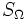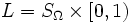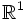# Long line

This article describes a standard counterexample to some plausible but false implications. In other words, it lists a pathology that may be useful to keep in mind to avoid pitfalls in proofs
View other standard counterexamples in topology

## Definition

The long line is defined as follows: Let$S_\Omega$ denote the minimal uncountable well-ordered set. Then$L = S_\Omega \times [0,1)$, in the dictionary order, is the long line.

## Topological space properties

### Properties it does satisfy

• Linearly orderable space: It is defined using a linear order, so it is clearly linearly orderable
• Locally Euclidean space: It is in fact locally homeomorphic to$\R^1$
• Normal space

Thus the long line fails to satisfy only the second condition for a manifold; it is simply too long.